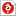검색어 입력폼

computer organization and architecture designing for performance 연습문제 10-12

저작시기 2017.12 |등록일 2019.03.31한컴오피스 (hwp) | 3페이지 | 가격 1,000원

목차

1. Convert the decimal number (45.125) to binary and hexadecimal number. Convert the binary number (11001.1111) to decimal and hexadecimal number.
2. Assume numbers are represented in 5-bit 2’s complement representation. Show the calculation of the following.
3. Express the following numbers in IEEE 32bit floating-point format.
4. What is the equivalent decimal value of the following IEEE 32 bit floating-point representation.
5. A given processor has words of 2bytes. What is the smallest and largest integer that can be represented in the following representations.
6. Show the calculation of adding +8 and –8. Assume numbers are represented in 8-bit 2’s complement representation. Show the following flags after the addition.
7. For the following data structure, draw the big-endian and little-endian layouts,and comment on the results.
8. List three possible places for storing the return address for a Procedure call.
9. How many different numbers can be represented in the IEEE 32bit floating-point representation.

본문내용

1.Convert the decimal number (45.125) to binary and hexadecimal number.
Convert the binary number (11001.1111) to decimal and hexadecimal number.
1. binary : hexadecimal : 0x
2. decimal : hexadecimal : 0x

2. Assume numbers are represented in 5-bit 2’s complement representation. Show the calculation of the following:
1) 6 + 10 00110 + 01010 = 10000 (Overflow) = -16
2) -6 + 10 11010 + 01010 = 00100 = 4
3) 6 – 10 00110 + 10110 = 11100 = -4
4) -6 – 10 11010 + 10110 = 10000 = -16

없음